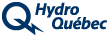Electricity Consumption

# Meter

Every time you use an electrical appliance, you're consuming power. Since there's a cost to consuming this power, meters record the exact amount of electricity (kilowatthours) that flows into a building. Hydro-Québec's power system stops at the electricity meter in your home.If the needle is between two numbers, take the smaller number. If it points between 9 and 0, take the 9. The reading for the current period is: 7,523 The reading for the previous period was: - 7,300 The difference between the two is: = 223 This difference, multiplied by 10, gives: 2,230 2,230 is the number of kilowatthours that will be billed.